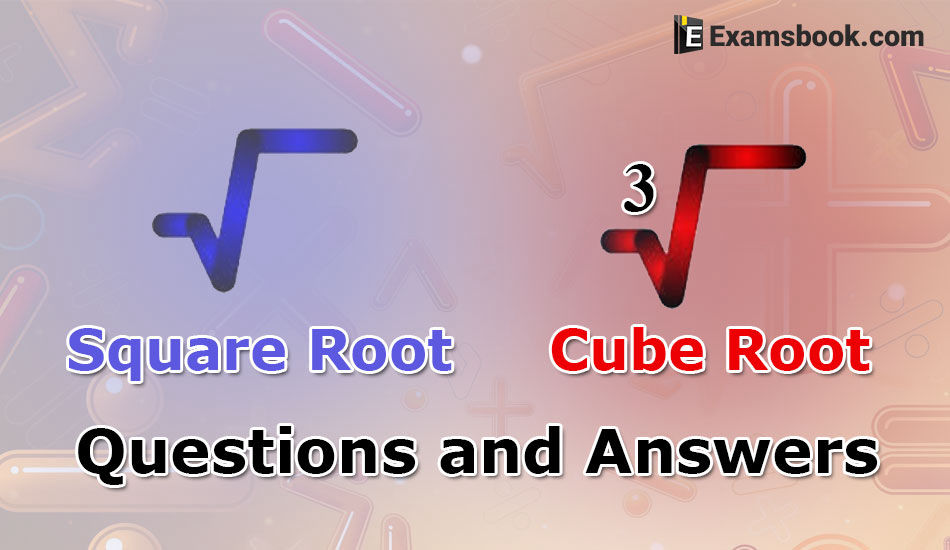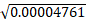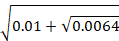• Save

# Square Root and Cube Root Questions and AnswersSquare root and Cube root questions have been asked in many competitive exams and there are strong chances to ask square root and cube root aptitude questions again in SSC, Bank and other competitive exams. Students should prepare for exams by practicing Square roots questions and cube root questions with answers.

Square root problems and Cube root questions are important and 1-2 questions asked in exams every year, try to solve square root questions & Cube root aptitude questions and answers for better results in upcoming competitive exams.

Visit here for more practice square root and cube root problems with solutions. If you want, you can more practice Cube Questions and Answers for obtaining good marks in competitive exams.

## Important Square Root and Cube Root Aptitude Questions:

1. The digit in the unit’s place in the square root of 15876 is:

(A) 1

(B) 3

(C) 6

(D) 9

Ans .   C

2. What is the square root of 0.16?

(A) 0.004

(B) 0.04

(C) 0.4

(D) 0.48

Ans .   C

3.equals:

(A) 0.000690

(B) 0.0069

(C) 0.0609

(D) 0.069

Ans .   B

4.=?

(A) 0.03

(B) 0.3

(C) 0.42

(D) None of these

Ans .   B

5. The least perfect square number divisible by 3,4,5,6 and 8 is:

(A) 700

(B) 1400

(C) 3500

(D) 3600

Ans .   D

6. The least number by which 294 must be multiplied to make it a perfect square, is:

(A) 3

(B) 5

(C) 6

(D) 24

Ans .   C

7. The least number by which 1470 must be divided to get a number which is a perfect square, is:

(A) 5

(B) 10

(C) 20

(D) 30

Ans .   D

8. What is the least number which should be subtracted from 0.000326 to make it a perfect square?

(A) 0.000002

(B) 0.0000016

(C) 0.0002

(D) 0.002

Ans .   A

Ask me in the comment section, if you face any problem while solving square root & cube root questions and answers. Visit next page for more Square Root and Cube Root questions and answers.